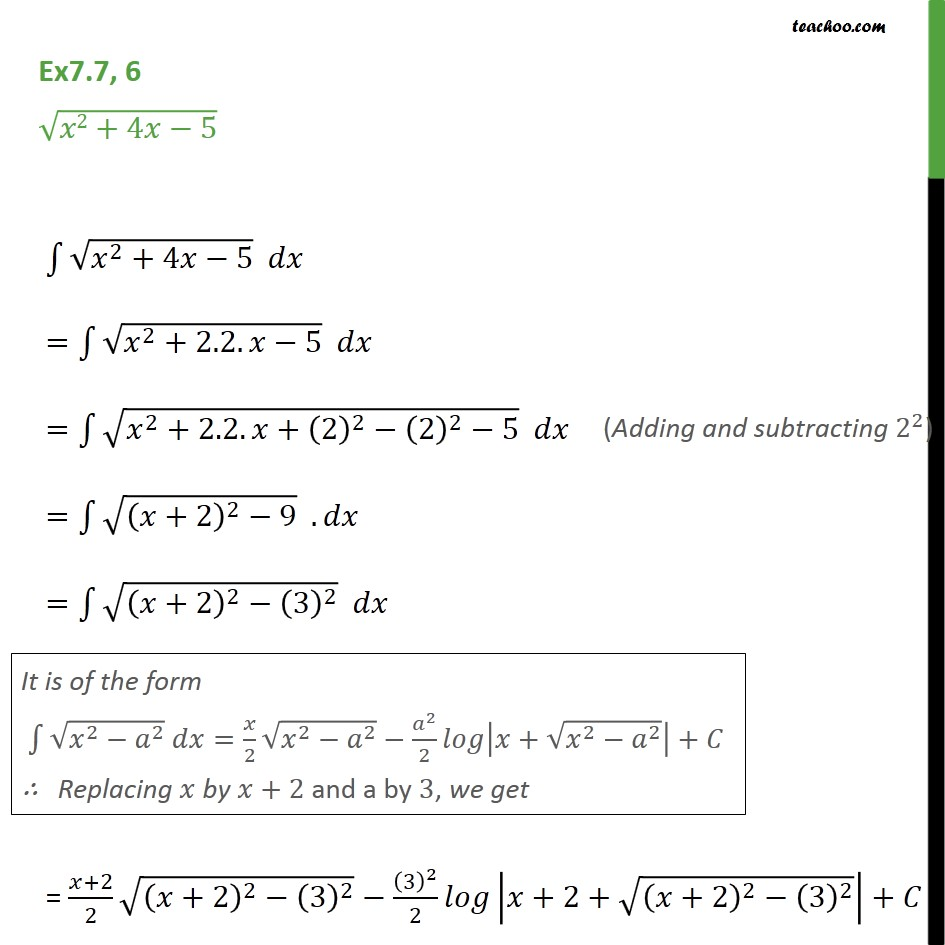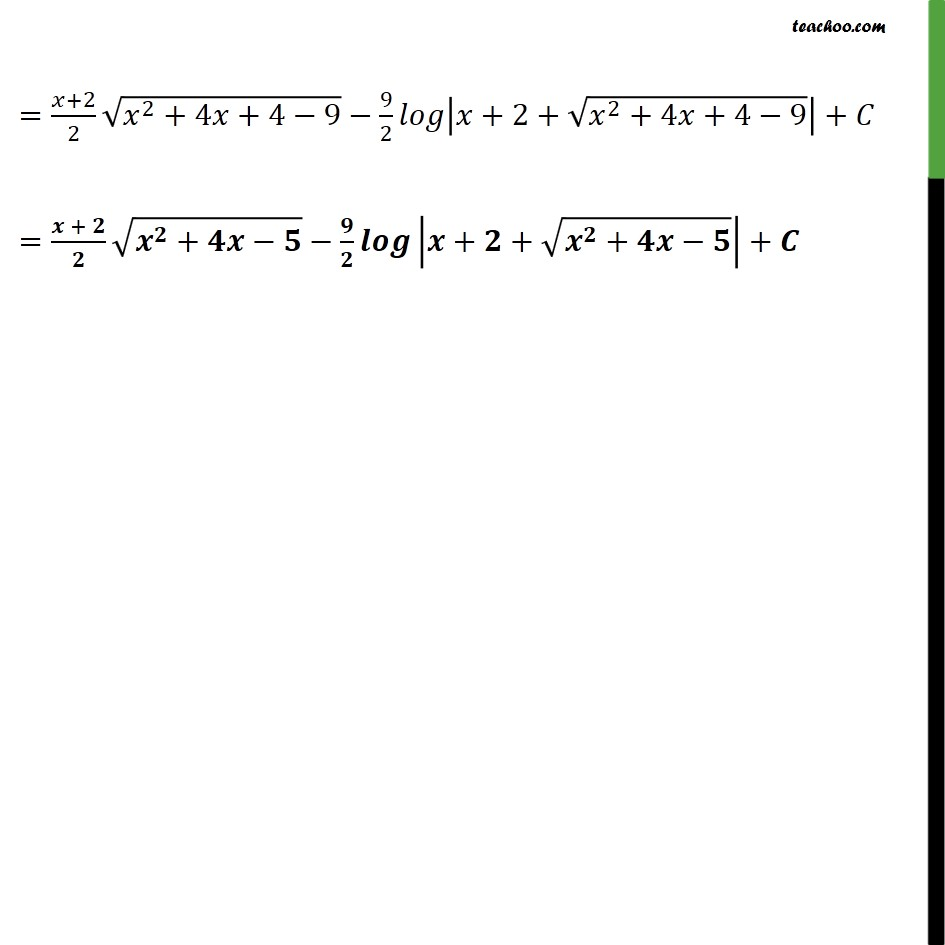Integration by specific formulaes - Formula 7

Chapter 7 Class 12 Integrals
Concept wiseLearn in your speed, with individual attention - Teachoo Maths 1-on-1 Class

### Transcript

Ex 7.7, 6 - Chapter 7 Class 12 Integrals - NCERT Integrate √(x^2 + 4x − 5) ∫√(x^2 + 4x − 5) dx = ∫√(x^2 + 2.2.x − 5) dx Adding and subtracting 2^2 = ∫√(x^2 + 2.2.x +(2)^2 - (2)^2 − 5) dx = ∫√((x + 2)^2 - 9) dx = ∫√((x + 2)^2 - 3^2) dx It is of the form ∫√x^2 - a^2 dx = x/2 √x^2 - a^2 - a^2/2 log |x + √x^2 - a^2| + C Replacing x by x + 2. a by 3 = (𝑥+2)/2 √(𝑥^2+4𝑥+4−9)−9/2 𝑙𝑜𝑔|𝑥+2+√(𝑥^2+4𝑥+4−9)|+𝐶 =(𝒙 + 𝟐)/𝟐 √(𝒙^𝟐+𝟒𝒙−𝟓)−𝟗/𝟐 𝒍𝒐𝒈|𝒙+𝟐+√(𝒙^𝟐+𝟒𝒙−𝟓)|+𝑪